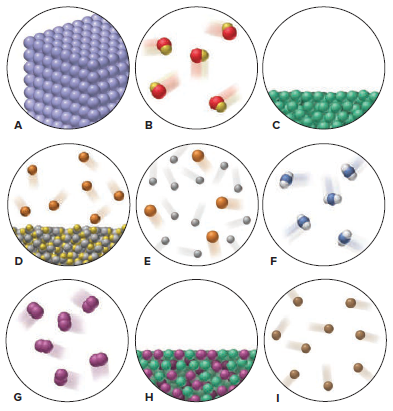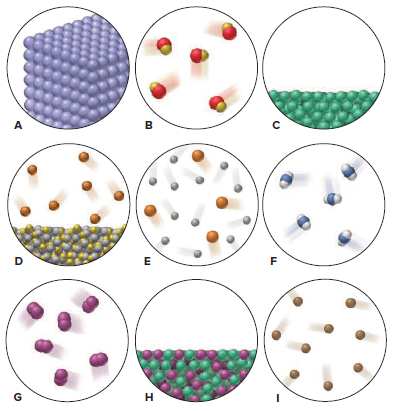Problem: Scenes A–I on the next page depict various types of matter on the atomic scale. Choose the correct scene(s) for each of the following:(a) A mixture that fills its container(b) A substance that cannot be broken down into simpler ones(c) An element with a very high resistance to flow(d) A homogeneous mixture(e) An element that conforms to the walls of its container and displays an upper surface(f) A gas consisting of diatomic particles(g) A gas that can be broken down into simpler substances(h) A substance with a 2/1 ratio of its component atoms(i) Matter that can be separated into its component substances by physical means(j) A heterogeneous mixture(k) Matter that obeys the law of definite composition

FREE Expert Solution
83% (282 ratings)
FREE Expert Solution

We are given the various types of matter depicted on the atomic scale:We are asked to choose from the given statements that best describe each depiction.

83% (282 ratings)
Problem Details

Scenes A–I on the next page depict various types of matter on the atomic scale. Choose the correct scene(s) for each of the following:

(a) A mixture that fills its container

(b) A substance that cannot be broken down into simpler ones

(c) An element with a very high resistance to flow

(d) A homogeneous mixture

(e) An element that conforms to the walls of its container and displays an upper surface

(f) A gas consisting of diatomic particles

(g) A gas that can be broken down into simpler substances

(h) A substance with a 2/1 ratio of its component atoms

(i) Matter that can be separated into its component substances by physical means

(j) A heterogeneous mixture

(k) Matter that obeys the law of definite compositionWhat scientific concept do you need to know in order to solve this problem?

Our tutors have indicated that to solve this problem you will need to apply the Law of Definite Proportions concept. You can view video lessons to learn Law of Definite Proportions Or if you need more Law of Definite Proportions practice, you can also practice Law of Definite Proportions practice problems .

How long does this problem take to solve?

Our expert Chemistry tutor, Rae-Anne took 7 minutes to solve this problem. You can follow their steps in the video explanation above.

What professor is this problem relevant for?

Based on our data, we think this problem is relevant for Professor Foster's class at GMU.

What textbook is this problem found in?

Our data indicates that this problem or a close variation was asked in . You can also practice practice problems .